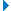## Overall Survey Results:

Text Block:
Please give your honest and fair assessment of the performance of all the players who took part in this game, including playing substitutes. I would prefer that those who complete this function have watched 90 minutes of the game in question.Don't forget to grade those players mentioned in the second section below.
1Here are the first ten team members:-

1 = 1 , 2 = 2 , 3 = 3 , 4 = 4 , 5 = 5 , 6 = 6 , 7 = 7 , 8 = 8 , 9 = 9 , 10 = 10
 1 2 3 4 5 6 7 8 9 10
Number of
Responses
Rating
Score*
Lloris 166 6.6
Aurier 166 7.5
Alderweireld 166 7.0
Dier 166 6.5
Reguilon 166 6.5
Hojbjerg 166 6.8
Lo Celso 166 6.3
Bale 166 8.8
Dele 166 6.6
Son 166 7.4
*The Rating Score is the weighted average calculated by dividing the sum of all weighted ratings by the number of total responses.Show Details
2Don't forget to grade these players too! Note that up to five substitutes are allowed for the remainder of this season, but they have to be made on no more than three occasions.

1 = 1 , 2 = 2 , 3 = 3 , 4 = 4 , 5 = 5 , 6 = 6 , 7 = 7 , 8 = 8 , 9 = 9 , 10 = 10
 1 2 3 4 5 6 7 8 9 10
Number of
Responses
Rating
Score*
Kane 166 6.2
Winks 165 5.4
Bergwijn 163 5.7
Lamela 162 5.5
*The Rating Score is the weighted average calculated by dividing the sum of all weighted ratings by the number of total responses.Show Details
3I would also like you to give marks out of 10 in the following skill areas:-

1 = 1 , 2 = 2 , 3 = 3 , 4 = 4 , 5 = 5 , 6 = 6 , 7 = 7 , 8 = 8 , 9 = 9 , 10 = 10
 1 2 3 4 5 6 7 8 9 10
Number of
Responses
Rating
Score*
SKILL 159 7.2
PLUCK (EFFORT) 159 7.3
UNFORCED ERRORS (The less errors, the better the mark) 158 6.9
RESOLVE (Defensive ability) 159 7.1
SCORE RATIO (Goals scored in relation to shots) 159 7.0
*The Rating Score is the weighted average calculated by dividing the sum of all weighted ratings by the number of total responses.Show Details
4
Now please grade Ryan Mason on three areas - Team Selection, Tactics, and Substitutions

1 = 1 , 2 = 2 , 3 = 3 , 4 = 4 , 5 = 5 , 6 = 6 , 7 = 7 , 8 = 8 , 9 = 9 , 10 = 10
 1 2 3 4 5 6 7 8 9 10
Number of
Responses
Rating
Score*
Team Selection 163 7.8
Tactics 162 7.7
Substitutions 163 6.7
*The Rating Score is the weighted average calculated by dividing the sum of all weighted ratings by the number of total responses.Show Details
5Finally, here's a chance for you to give a single mark out of ten for the performance of the officials, led by referee Andre Marriner.  (VAR has to be considered part of your considerations).

1 = 1 , 2 = 2 , 3 = 3 , 4 = 4 , 5 = 5 , 6 = 6 , 7 = 7 , 8 = 8 , 9 = 9 , 10 = 10
 1 2 3 4 5 6 7 8 9 10
Number of
Responses
Rating
Score*
161 4.7
*The Rating Score is the weighted average calculated by dividing the sum of all weighted ratings by the number of total responses.Show Details